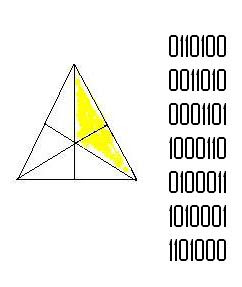## M Theory Lesson 80

Recall that 3×3 complex circulants are useful for building mass operators. A basic 7×7 circulant is the incidence matrixfor the plane shown. This matrix is also a generator matrix  for the linear binary Hamming code which contains 16 binary words of length 7. The error correction for the Hamming code works by adjoining three bits $x_5, x_6$ and $x_7$ to an element $(x_1, x_2, x_3, x_4)$ of $\mathbb{F}_{2}^{4}$ such that if one labels the Fano points by the $x_{i}$, with the error bits at the centre of the outer edges, the sum of four vertices on the edge triangles equals zero. For example, a codeword 1000110 satisfies

$x_1 + x_2 + x_4 + x_6 = 1 + 0 + 0 + 1 = 0$

The weight 3 codewords appear in the matrix above. This says that PSL(2,7) is isomorphic to GL(3,2), the automorphism group of the projective plane on $\mathbb{F}_{2}$.

We saw this a while ago while pondering octonion products and their possible relation to non-associative triple ribbon diagrams. Any binary code gives a lattice via the group homomorphism $\mathbb{Z}^{n} \rightarrow \mathbb{F}_{2}^{n}$ for which the inverse image of a code defines a lattice. For convenience one multiplies the lattice coordinates by a factor of $\sqrt{2}^{-1}$. Thus there is a way to assign a theta series to any binary code. By adding a check bit to the Hamming code (an eighth bit which is the sum of the others) one obtains a code which maps to an even unimodular lattice in $\mathbb{R}^{8}$, otherwise known as the E8 lattice.

 Lattices and Codes, W. Ebeling (1994) (based on lectures by F. Hirzebruch)

## 2 Responses so far »

1. 1### L. Riofrio said,

Another great post on M-theory. I hope the world appreciates your skill in maths. We definitely need more good mathematicians.

Black Holes can exist without galaxies, but every galaxy yet found has at its centre a Black Hole. People can’t figure out that BH’s precede galaxies?

2. 2### Kea said,

Hi Louise! Yes, people are being very stubborn and stupid.

I just went to a seminar by D. Wiltshire which showed that his varying-clock no-DE cosmology satisfies several tests much better than LambdaCDM, such as a best fit Hubble parameter around 62 in agreement with recent measurements.